# Integration by parts

• Introduction

• The method

• 18 Resolved Integrals by parts

## Introduction

When the integrand is formed by a product (or a division, which we can treat like a product) it's recommended the use of the method known as integration by parts, that consists in applying the following formula: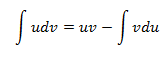Even though it's a simple formula, it has to be applied correctly. Let's see a few tips on how to apply it well:

• Select u and dv correctly: A bad choice can complicate the integrand. Supposing we have a product, and one of the factors is monomial (x3 for example). If we consider that dv = x3, then by using integration we obtain that

$$v = \frac{x^4}{4}$$

We have increased the exponent and this could mean a step back in the process.

Something similar happens with fractions (like 1/x). If we take dv = 1/x, we will obtain v = log|x|, and probably end up with a harder integration process.

As a rule, we will call u all powers and logarithms; and dv exponentials, fractions and trigonometric functions (circular functions).

• Don't change our minds about the selection: Sometimes we need to apply the method more than once for the same integral. When this happens, we need to call u the result of du from the first integral we applied the method to. The same applies to dv. If we don't do this, seeing as choosing one option or another involves integration or differentiating, we'll be undoing the previous step and we won't be able to advance.

• Cyclic integrals: Sometimes, after applying integration by parts twice we have to isolate the very integral from the equality we've obtained in order to resolve it. An example of this is exercise 10.

# Integrals solved step by step

Integral 1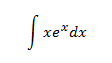Show solution

Integral 2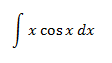Show solution

Integral 3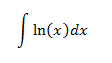Show solution

Integral 4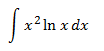Show solution

Integral 5Show solution

Integral 6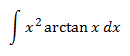Show solution

Integral 7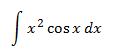Show solution

Integral 8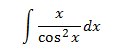Show solution

Integral 9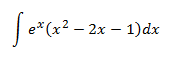Show solution

Integral 10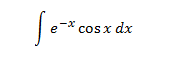Show solution

Integral 11Show solution

Integral 12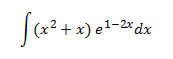Show solution

Integral 13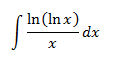Show solution

Integral 14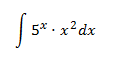Show solution

Integral 15Show solution

Integral 16Show solution

Integral 17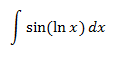Show solution

Integral 18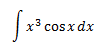Show solution